Sie sind hier: ICP » R. Hilfer » Publikationen

# 1 Introduction

[page 637, §1]
[637.1.1] A special function of growing importance is the generalized Mittag-Leffler function defined by the power series [2, p. 210]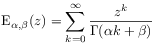(1)

for complex argument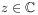and parameters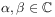with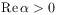. [637.1.2] Despite the fact that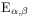was introduced roughly 100 years ago [3, 4, 5, 6, 7, 8] its mapping properties in the complex plane are largely unknown.

[637.2.1] Mittag-Leffler functions are important in mathematical as well as in theoretical and applied physics [9, 10, 11, 12, 13, 14, 15, 16, 17, 18]. [637.2.2] A primary reason for the recent surge of interest in these functions [page 638, §0]    is their appearance when solving the fractional differential equation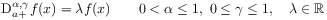(2)

where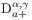is a fractional derivative of order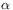and type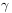with lower limit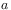. [638.0.1] In eq. (2) the symbolstands for [18, p. 115]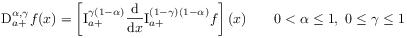(3)

for functions for which the expression on the right hand side exists. [638.0.2] Of course, the notation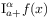stands for the right sided fractional Riemann-Liouville integral of order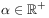defined by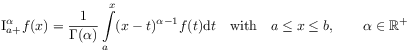(4)

for locally integrable functions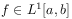. [638.0.3] Recall from Ref. [18, p. 115] that equation (2) is solved for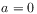by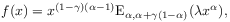(5)

where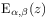is the generalized Mittag-Leffler function. [638.0.4] Equation (2) shows that the Mittag-Leffler function plays the same role for fractional calculus that the exponential function plays for conventional calculus. [638.0.5] Mittag-Leffler functions and fractional calculus have in recent years become a powerful tool to investigate anomalous dynamics and strange kinetics [13, 18, 19, 20].

[638.1.1] Despite the growing importance ofin physics, and despite a wealth of analytical information aboutits behaviour as a holomorphic function and dependence upon the parameters are largely unexplored, because there seem to be no numerical algorithms available to compute the function accurately for all,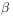,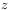. [638.1.2] Easy numerical evaluation and a thorough understanding ofas a function of,,is, however, a key prerequisite for extending its applications to other disciplines. [638.1.3] It is therefore desirable to explore the behaviour offor large sets of the parameters,and complex argument.

[638.2.1] Given this objective the present paper reports a newly developed numerical algorithm as well as extensive computations for the generalized Mittag-Leffler function. [638.2.2] Little will be said in this paper about the algorithm apart from giving its complete definition a (This is a footnote:) aWhile this work was in progress a simpler algorithm appeared in . A detailed comparison between the two algorithms can be found in .. [638.2.3] One should note that the algorithm works not only on the real axis, but in the full complex plane. [638.2.4] Rather than discussing details of the algorithm we concentrate here on exploring the functional behaviour of. [638.2.5] In particular we study its complex zeros and illustrate its behaviour as an entire function. [638.2.6] As an example we find that the zeros ofcoalesce to form a simple pole in the limit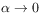. [638.2.7] Moreover, the zeros diverge in a complicated fashion to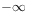asapproaches unity from above as well as from below.